## research papersJOURNAL OFSYNCHROTRONRADIATION
ISSN: 1600-5775
Volume 26| Part 1| January 2019| Pages 215-219

## Single-image phase retrieval for hard X-ray grating interferometry

aSchool of Electronic Science and Applied Physics, Hefei University of Technology, Anhui 230009, People's Republic of China, bBeijing Advanced Innovation Center for Imaging Technology, Capital Normal University, Beijing 100048, People's Republic of China, cShanghai Synchrotron Radiation Facility, Shanghai Institute of Applied Physics, Chinese Academy of Sciences, Shanghai 201204, People's Republic of China, and dNational Synchrotron Radiation Laboratory, University of Science and Technology of China, Anhui 230026, People's Republic of China
*Correspondence e-mail: dywangzl@hfut.edu.cn

(Received 8 August 2018; accepted 24 November 2018)

A single-image method is proposed for quantitative phase retrieval in hard X-ray grating interferometry. This novel method assumes a quasi-homogeneous sample, with a constant ratio between the real and imaginary parts of its complex refractive index. The method is first theoretically derived and presented, and then validated by synchrotron radiation experiments. Compared with the phase-stepping method, the presented approach abandons grating scanning and multiple image acquisition, and is therefore advantageous in terms of its simplified acquisition procedure and reduced data-collection times, which are especially important for applications such as in vivo imaging and phase tomography. Moreover, the sample's phase image, instead of its first derivative, is directly retrieved. In particular, the stripe artifacts encountered in the integrated phase images are significantly suppressed. The improved quality of the retrieved phase images can be beneficial for image interpretation and subsequent processing. Owing to its requirement for a single image and its robustness against noise, the present method is expected to find use in potential investigations in diverse applications.

### 1. Introduction

Over the last two decades, X-ray phase-contrast imaging has been studied intensively, mainly owing to its improved contrast for weakly attenuating samples, such as biomedical soft tissues with small attenuation differences (Snigirev et al., 1995; Pfeiffer et al., 2006; Bravin et al., 2013). Among various X-ray phase-contrast imaging techniques, X-ray grating interferometry (XGI) has demonstrated its unique ability to be fully compatible with conventional X-ray tubes (Pfeiffer et al., 2006). XGI can provide the absorption, refraction (i.e. differential phase) and scattering properties of a sample simultaneously, and therefore has shown significant promise in diverse applications (Wang, Hauser et al., 2014; Sarapata et al., 2015; Eggl et al., 2015).

In XGI, the acquired sample images can contain a mixture of absorption and refraction contrast. Many methods have been developed for the separation and evaluation of the two quantities (Weitkamp et al., 2005; Momose et al., 2009; Wang et al., 2013; Pelliccia et al., 2013; Ge et al., 2014; Wang, Gao et al., 2014). Recently, several retrieval methods making use of a single image have been proposed for XGI (Momose et al., 2009; Ge et al., 2014; Wang, Gao et al., 2014). Compared with the phase-stepping method (Weitkamp et al., 2005), such a method would be preferable to shorten the acquisition time and minimize the effects of possible sample movements, a crucial requirement in many applications such as dynamic imaging (Momose et al., 2011). Furthermore, quantitative analysis and subsequent image processing require direct retrieval of the sample's phase image, rather than its first derivative. Although in principle the phase image can be obtained by the integration of the refraction image (Hasnah et al., 2005), in practice this procedure very often fails to provide phase images of satisfactory quality (Kottler et al., 2007). This has been a well known problem in both X-ray analyzer-based imaging and XGI, and several approaches have been developed in order to alleviate this effect (Wernick et al., 2006; Thüring et al., 2011; Nilchian et al., 2016).

In this work, we propose a novel method for data acquisition and direct retrieval of the sample's phase image from a single projection image in XGI. This method takes advantage of the fact that a quasi-homogeneous sample has a constant ratio between the real and imaginary parts of its complex refractive index. The phase-retrieval formula is theoretically derived and presented, and then validated by synchrotron radiation experiments. The results demonstrate that the proposed method provides quantitative phase images with improved quality, and is highly stable with respect to noise.

### 2. Single-image method for phase retrieval

Fig. 1(a) shows a typical X-ray grating interferometer setup for parallel-beam geometry. Through the fractional Talbot effect (Weitkamp et al., 2005), a self-image pattern is generated at specific distances downstream of the phase grating G1. With a sample positioned in front of G1, the self-image pattern is locally distorted, including attenuation in mean intensity, local shift of the patterns and loss of fringe visibility. An absorption grating G2 with the same period as the self-image pattern, superimposed on the distorted self-image, acts as an analyzer to convert the distortion into intensity variations recorded by the detector. Scanning one of the gratings in the x direction leads to the intensity curve L [shown in Fig. 1(b)] that describes how the measured intensity changes as a function of the relative displacement xg between the two gratings. With the sample in place and xg = −p2/4, the intensity measured by the detector, ID, can be written as (Wang et al., 2013; Wang, Gao et al., 2014)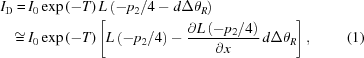where I0 is the incident intensity on the sample, p2 is the grating period, T =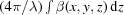represents the sample's absorption, with λ being the X-ray wavelength, d is the distance between the gratings G1 and G2,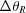=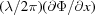is the X-ray beam's refraction, with Φ =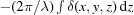being the X-ray beam's phase shift induced by the sample, and n = 1 − δ + iβ is the sample's complex refractive index. Note that in the derivation of the second line of equation (1), we assumed that the local shift dΔθR due to the sample's refraction is small compared with p2/4, so that a first-order Taylor expansion can safely be adopted for the intensity curve on one of its slopes, as shown in Fig. 1(b).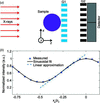Figure 1 (a) A schematic representation of the X-ray grating interferometer. (b) A plot of the intensity curve L as a function of xg in units of the grating period p2. The crosses correspond to the measured values, while the solid line shows a sinusoidal fit and the dashed line the linear approximation around xg = −p2/4.

As shown in equation (1), the image signal is dependent on both of the two unknowns T and ΔθR, which in turn depend on the spatial distributions of β and δ. The logarithm of equation (1)is given by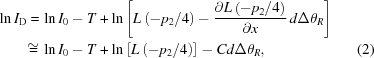with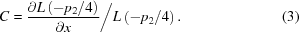For further analysis, we consider the assumption that the ratio γ = δ/β is approximately constant across the sample, so that Φ = −γT/2. Strictly speaking, this assumption is only valid for samples composed of a single material. However, it has been shown that this approximation can also provide good results in many practical cases (Paganin et al., 2002; Pavlov et al., 2004; Briedis et al., 2005; Weitkamp et al., 2011; Diemoz et al., 2015). In particular, this approximation is well suited for the imaging of soft biological tissues with quite similar chemical compositions (Olendrowitz et al., 2012; Wernersson et al., 2013; Astolfo et al., 2016). Under this assumption, equation (2)can then be rewritten as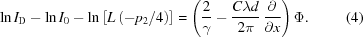Now we take the one-dimensional Fourier transform of equation (4)and, by use of the Fourier derivative theorem, we obtain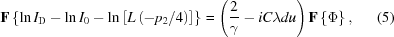where F denotes the one-dimensional Fourier transform and u is the Fourier space coordinate. From equation (5), it is obvious that a single image ID as input is sufficient for retrieval of the sample's phase image,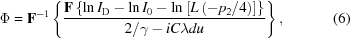where F−1 indicates the inverse one-dimensional Fourier transform. Under the assumption of a constant δ/β ratio, a similar expression for the sample's absorption T can also be obtained,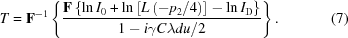### 3. Experimental validation

The novelty of the proposed method lies in its ability to enable the direct retrieval of the sample's phase image by the use of only a single intensity measurement. In order to test its validity, synchrotron radiation experiments were performed on beamline BL13W1 of the Shanghai Synchrotron Radiation Facility (SSRF). The grating interferometer consisted of a phase grating G1 that had a period of 2.396 µm and introduced a phase shift of π/2 for the design energy of 20 keV, and an analyzer grating G2 with a period of 2.4 µm and made of gold. The inter-grating distance was set to 4.65 cm, i.e. the first fractional Talbot distance. The detector, placed ∼7 cm downstream from the analyzer grating G2, was a scientific complementary metal–oxide–semiconductor (sCMOS) detector (Hamamatsu ORCA-Flash 4.0 V2) with a native pixel size of 6.5 µm. A 2 × 2 binning mode was used in the data acquisitions. The image acquired with the relative displacement xg set to −p2/4 is used to retrieve the phase image directly [equation (6)]. The exposure time is 6 ms. The sample used was a plastic cylinder made of acrylo­nitrile buta­diene styrene (ABS). It has a diameter of 3 mm and was placed 18 cm upstream of the phase grating G1. For calculations of the theoretical values of the complex refractive index, the CXRO database was used (Henke et al., 1993).

Figs. 2(a)–2(c) show the refraction, integrated and directly retrieved phase images of the cylinder, respectively. The refraction image is obtained by a three-step phase-stepping procedure, with a total exposure time of 6 ms. For the calculation of Fig. 2(c), the following nominal values were used: δ = 4.93 × 10−7 and β = 2.71 × 10−10. Note that for a better visualization, the values in the phase images have been inverted. An improved image quality can be observed for the directly retrieved phase image, if Fig. 2(c) is compared with Fig. 2(b). In particular, the horizontal stripe artifacts, from which the phase image obtained by a simple integration of the refraction image suffers (Kottler et al., 2007; Thüring et al., 2011), are greatly suppressed. This can be mainly attributed to the additional low-pass filtering present in equation (6). For a quantitative evaluation, the standard deviation over a background area is calculated. The corresponding values are 0.9298 for the directly retrieved phase image and 1.8344 for the integrated phase image. This again confirms the improved image quality.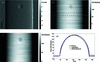Figure 2 (a) The refraction image of the cylinder sample, (b) the integrated phase image, (c) the directly retrieved phase image and (d) the horizontal line profile along the dashed line in panel (b). The scale bar = 0.65 mm.

Fig. 2(d) presents the horizontal line profile at the position indicated by the dashed line in Fig. 2(b), along with the theoretical prediction, to demonstrate the quantitative accuracy of the presented method. It can be seen that the phase shift retrieved through the single-image approach is a good approximation of the theoretically predicted values, apart from a slight blur at the edges and a small underestimation inside the cylinder. This inconsistency might result from inhomogeneities in the material and/or inexact values for the material's complex refractive index. In comparison, the profile obtained through a simple integration differs more from the theoretical prediction: the phase shift is overestimated within the cylinder and does not return to the baseline at the end of the cylinder, leading to a gross overestimation outside it. This can be explained by the accumulation of errors in the refraction image during the integration.

Fig. 3(a) shows the absorption image of the cylinder sample, and the line profile along the dashed line is shown in Fig. 3(b), together with the theoretical prediction. The agreement of the two line profiles is quantitatively excellent, confirmed by the value of the calculated correlation coefficient R = 0.9973.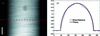Figure 3 (a) The directly retrieved absorption image of the cylinder sample and (b) the line profile along the dashed line in panel (a). The scale bar = 0.65 mm.

Finally, the method's robustness against noise was investigated. Fig. 4(a) shows the retrieved phase image by use of a single projection image with an exposure time of only 2 ms, while Fig. 4(b) presents the difference between the retrieved phase images obtained with low [Fig. 2(c)] and high [Fig. 4(a)] levels of noise. As can be seen, despite the decreased exposure time (i.e. increased noise level) of the input image, the retrieved phase image still remains in agreement with that from a high photon count, supported by the fact that the difference image shown in Fig. 4(b) has a mean value of only 0.9735. This high stability with respect to noise, as seen from Figs. 4(a) and 4(b), can be understood by the fact that equation (6)actually acts as a low-pass filter, which has the effect of significantly suppressing high-frequency noise in the image. Meanwhile, the filter never diverges at low frequencies, and therefore we expect equation (6)also to be well behaved at low frequencies. Finally, it is worth mentioning that this property is a direct result of exploiting both absorption and refraction information from the input projection image (Diemoz et al., 2015).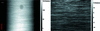Figure 4 (a) The directly retrieved phase image with an exposure time of 2 ms and (b) the difference between the phase images in Figs. 2(c) and 3(a) (note the different grayscales used). The scale bar = 0.65 mm.

### 4. Conclusions

A single-image method has been proposed for quantitative phase retrieval in X-ray grating interferometry (XGI). This novel method makes the assumption that the ratio δ/β is approximately constant across the sample. The phase-retrieval formula has been derived theoretically and then tested on synchrotron radiation experiments. The results demonstrate that with a priori knowledge of the ratio δ/β, this new method can provide quantitative phase images with improved quality and suppression of the stripe artifacts often encountered with differential phase-contrast techniques. This aspect is crucial for improving the analysis of structures in examined materials, e.g. in medical images, and the computation of mass-density images (Wernick et al., 2006).

It has also been shown that this method is quite robust with respect to noise, owing to its low-pass filtering property. Because of the requirement for only a single input image, this method is advantageous in terms of reduced exposure time and potentially decreased dose delivered to the sample. Therefore, we can expect a widespread application of the new method in areas where exposure time and/or radiation dose are critical, such as dynamic imaging (Momose et al., 2011).

Future work will be dedicated to the extension of the method to the case of multi-material samples (Beltran et al., 2010; Zamir et al., 2017). Finally, the developed method can be directly generalized to two-dimensional XGI (Sato et al., 2011; Morimoto et al., 2015) and also applied to phase tomography (Ruiz-Yaniz et al., 2016).

### Funding information

The following funding is acknowledged: National Natural Science Foundation of China (grant Nos. U1532113, 11475170, 11505188, 11205157); Natural Science Foundation of Anhui Province (grant No. 1508085MA20).

### ReferencesJOURNAL OFSYNCHROTRONRADIATION